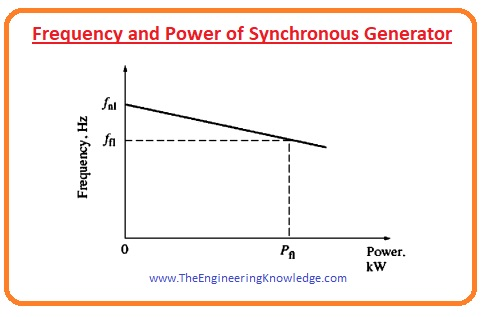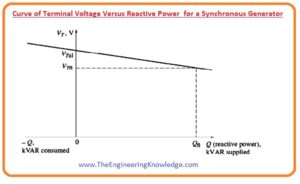Hello friends, I hope all of you are fine. In today’s tutorial, we are gonna have a look at Frequency-Power and Voltage-Reactive Power Characteristics of a Synchronous Generator. Synchronous generator first time was introduce to world in 1870 by P.N Jablochkov. These generators are used in submissions where continuous speed is desired. The operation of this device is very modest as we know it is not self-starting machine. An exterior dc source is coupled with its rotor when rotor is revolved by prime mover than rotating magnetic field is produced in its stator. Instead of external source permanent magnet rotor can also use for it but mostly it connected with small generator or exciters.

In today’s post, we will have a look at its output frequency and power relationship and also discuss relation between reactive power and generated a voltage. So let’s get started with Frequency-Power and Voltage-Reactive Power Characteristics of a Synchronous Generator.

#### Frequency-Power and Voltage-Reactive Power Characteristics of a Synchronous Generator

• Any type of generator either it is alternating current or direct current generator, gets mechanical energy from some external sources that are known as a prime mover.
• There are many categories of prime movers like steam turbines, hydro, wind, or gas turbines.
• It does not matter either which type of the prime mover is working on the generator its speed with which it rotates generator decreases when the load on the generator increases.
• To maintain the speed of the prime mover a speed limiter is introduced on the generator that called governor mechanism, it controls the speed of the prime mover to reduce, in case of any variation in the load or high-power demand.
• The governor adjusts the prime mover speed in such a way that it shows dropping characteristic (it is a curve of a generator that specifies a decrease in voltage when load increases on the generator use a high amount of power) with the increment in a load of generator.
• The speed drop equation of the prime mover is written as.

SD = (nnl – nfl) nfl x 100

#### Frequency and Power of Synchronous Generator

• The expression that explains the relation between the speed of the generator’s rotor and its stator’s frequency is given as.

fe = nmP/120

• In this equation the.
• fe is the speed of the frequency of the generator’s stator.
• nm is the rotation speed of the rotor.
• P is no of poles of the generator.
• The graphical representation of the frequency and power is drawn in given figure.• This kind of graphical representation of the frequency and power is very useful for the parallel operation of the generator.
• The quantitative relation of frequency and power can be written as.

P = SP(fnl – fsys)

• P is the output power of the synchronous generator.
• fnl is the frequency of the generator when there is no load at a shaft of the generator.
• fsys is the frequency of the system it can be 50 or 60.
• SP is the slop of the characteristic curve that is formed between the frequency and the power of the generator, and it measured in Kw/hz or Mw/hz.

#### Voltage and Reactive power of the Synchronous Generator

• The equation that we discussed can also make this type of relation between the reactive power and the terminal voltage of the generator.
• As we discussed in the synchronous generator operating alone article that when the load on the generator is inductive, there is a voltage loss at its output.
• When the load is capacitive the value of the output voltage VT
• The graphical representation among the terminal voltage and the reactive power is shown in a given figure, and it also shows the drooping characteristic of the synchronous generator.• We can see from the figure that the graph is a straight line, it is naturally not a straight line, it is due to voltage regulators. That makes it linear by maintaining the value of the voltage.
• The location of the curve can transfer up and down in the graph by changing the no-load voltage point location, it can be done by the voltage regulators.
• Like the power frequency, these characteristics (VT and Q) also play a very important rule in parallel operation of the synchronous generator.
• The relation among the VT and the Q can be written like the relation that we make between the power and frequency in given below equation if the output of the voltage regulator shows linear behavior with the variation in the reactive power (Q).

P = SP(fnl – fsys)

• The important point is that the when a generator is working lonely, the active power (P) and the (Q) generated by the generator depends on the load connected with the generator, both powers are not regulated by the governors of the generator.
• So, the frequency fe frequency and reactive power Q of the generator are controlled by the governor of the generator and the terminal voltage is controlled by the field current of the generator.

#### Conclusion

• From the above discussion, we can conclude some points that are described here.
• The active power P and reactive power Q generated by the generator depends on the quantity of the load connected with the generator.
• The governor of the generator controls the frequency of the system fe.
• The field current of the generator controls the output voltage VT of the generator.

You can also read some related topics to synchronous generator that are listed here.

Introduction to Synchronous Generator

Synchronous Generator Equivalent Circuit

Synchronous Generator Phasor Diagram

Synchronous Generator Power and Torque

Synchronous Generator Parameters

Synchronous Generator Operating Alone

Synchronous Generator Parallel Operation

Synchronous Generator parallel with Large Power system

Synchronous Generator Parallel with same Size Generator

Synchronous Generator Ratings

Synchronous Generator Capability Curves

Synchronous Generator Transients

That all about Frequency-Power and Voltage-Reactive Power Characteristics of a Synchronous Generator I have mentioned each and everything related to this topic. If you want to know something more can ask in comments. See you in the next tutorial.

### 1 Comment

1. jon says:

well written

2. Rickie Youngerman says:

Thanks for your article. I would love to say a health insurance brokerage also works for the benefit of the coordinators of any group insurance policy. The health agent is given an index of benefits wanted by anyone or a group coordinator. What any broker can is hunt for individuals or even coordinators that best match up those demands. Then he shows his recommendations and if each party agree, the actual broker formulates binding agreement between the two parties.